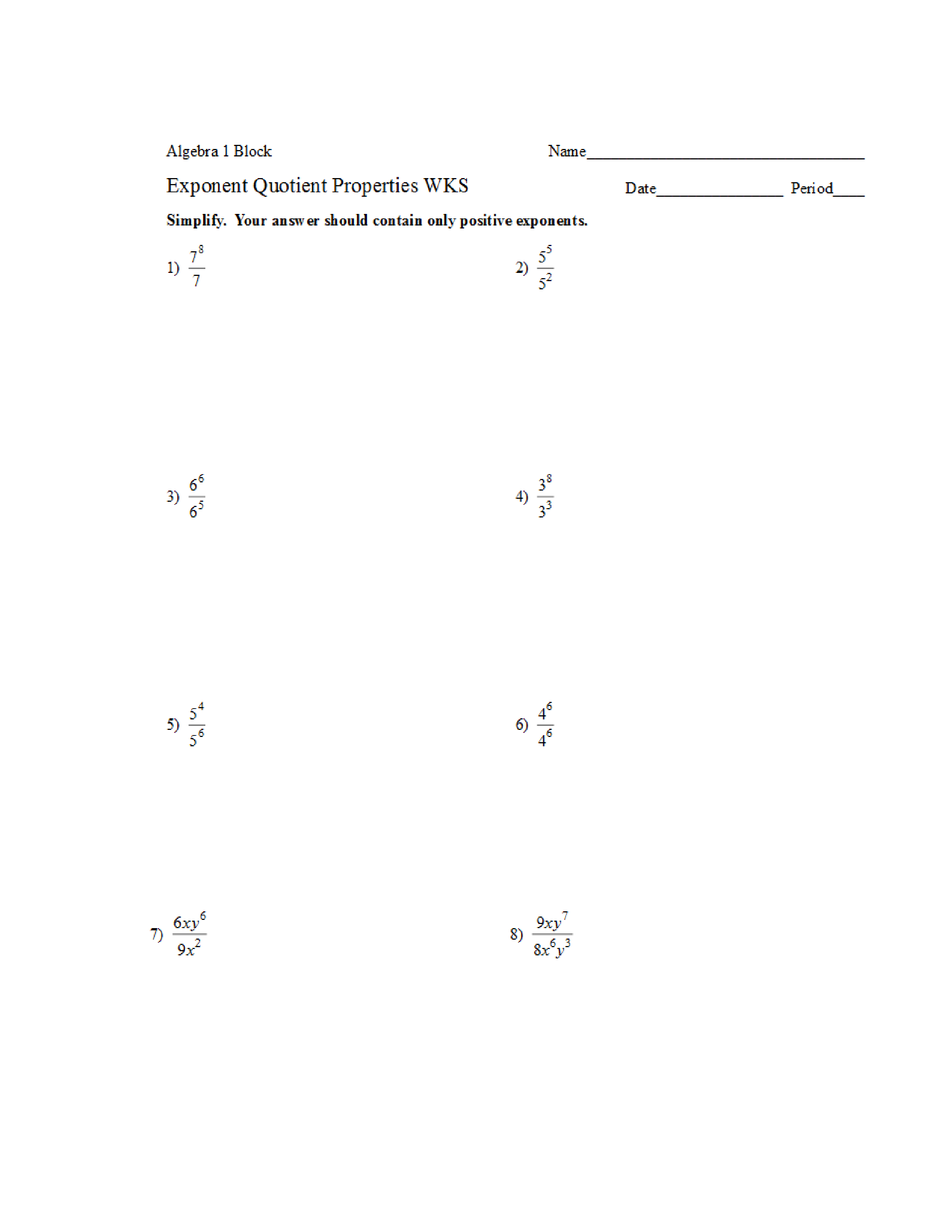Kindergarten
1st
2nd
3rd
4th
5th
6th
7th
8th
9th
10th
11th
12th
Higher Ed
Other
Subjects
ELA
Math
Science
Social Studies
Art
Computer Science
French
German
Music
Physical Education
Spanish
Other
Private Library
Exponent Quotient Properties Practice
starstarstarstarstarstarstarstarstarstar
by Nicholas Nenni
| 20 Questions
Note from the author:
Practicing applying quotient properties to exponents
zoom in1
1 pt
Simplify. Leave your answer with an exponent. Use / for a fraction
2
1 pt
Simplify. Leave your answer with an exponent. Use / for a fraction
3
1 pt
Simplify. Leave your answer with an exponent. Use / for a fraction
4
1 pt
Simplify. Leave your answer with an exponent. Use / for a fraction
5
1 pt
Simplify. Leave your answer with an exponent. Use / for a fraction
6
1 pt
Simplify. Leave your answer with an exponent. Use / for a fraction
7
1 pt
Simplify completely. Use / for a fraction
8
1 pt
Simplify completely. Use / for a fraction
zoom in9
1 pt
Simplify completely. Use / for a fraction
10
1 pt
Simplify completely. Use / for a fraction
11
1 pt
Simplify completely. Use / for a fraction
12
1 pt
Simplify completely. Use / for a fraction
13
1 pt
Simplify completely. Use / for a fraction
14
1 pt
Simplify completely. Use / for a fraction
15
1 pt
Simplify completely. Use / for a fraction
16
1 pt
Simplify completely. Use / for a fraction
zoom in17
1 pt
Simplify completely. Use / for a fraction
18
1 pt
Simplify completely. Use / for a fraction
19
1 pt
Simplify completely. Use / for a fraction
20
1 pt
Simplify completely. Use / for a fraction
Add to my formatives list